# Assignment 4: Reinforcement learning 1

*** Due date: Start of class in Week 5 ***

## Part 1: Simulate the bandit (2 points)

• Follow along with the notes to develop a function to simulate the bandit with a win probability of 40% for 100 trials
• Write a script to call your function
• Plot the simulated rewards, label your axes and make your plot look nice

## Part 2: Implement the simple averaging model (2 points)

• Follow along with the notes to implement the naive version of the averaging model based on taking the mean of all rewards (put this in a function and call it from a script)
• Plot the resulting value on the same plot as the rewards, label axes and make your plot look nice

## Part 3: Implement the prediction error version of the averaging model (2 points)

• Follow along with the notes to implement the prediction error version of the averaging model (put it in a function and call it from a script)
• Plot the resulting values and compare with the naive version of the model
• This is not in the notes: Compute the learning rate on each trial and make a separate figure to plot how the learning rate changes over time in this model.

## Part 4: Implement the constant learning rate model (2 points)

• Follow along with the notes to implement the model with a constant learning rate of α = 0.1, put it in a function and call it from a script
• Apply the model to the 100 rewards you generated in Part 1
• Plot the values over time and compare the values you get from the constant learning rate model with those that you get from the simple averaging model

## Part 5: Test the constant learning rate model (2 points)

• Follow along with the notes to implement the bait-and-switch case and plot how the constant learning rate and simple averaging models behave in this case
• This is not in the notes: Now compare how the constant learning rate model changes its behavior for different values of α in the bait-and-switch situation. Try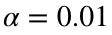,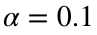, and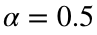.
• In the comments describe why you think the model behavior is changing.

## Part 6: Special cases for alpha (1 extra credit point)

The extra credit questions today involve Math, not coding. To hand in your work, just make a Word doc with equations in your Dropbox folder.
• Starting from the update equation for values in the fixed learning rate model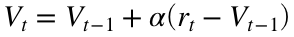, write down what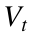is in terms of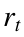and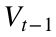when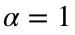• Now write down whatis in terms ofandwhen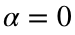• Interpret these results. What does they say about how learning rate changes learning? Can you square these findings with your simulations in the last question of Part 5.

## Part 7: Reinterpreting the constant learning rate model as a recency-weighted average of rewards (2 extra credit points)

Let's start from the equation for...What we are going to do is rewrite this in terms of a sum over all the rewardswhere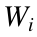is a weight that you are going to compute.
To do this we first take the expression for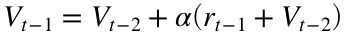and substitute it into the expression forto get ...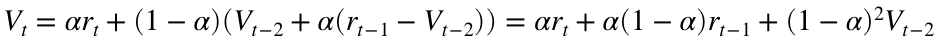Now, take the expression for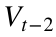and substitute it into this expression. Then do the same again for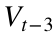. Hopefully by this point you can guess what the form ofis.
If you can guess the form ofuse Matlab to plot it as a function of i.
What does this mean in terms of howis computed from a weighted sum of rewards.